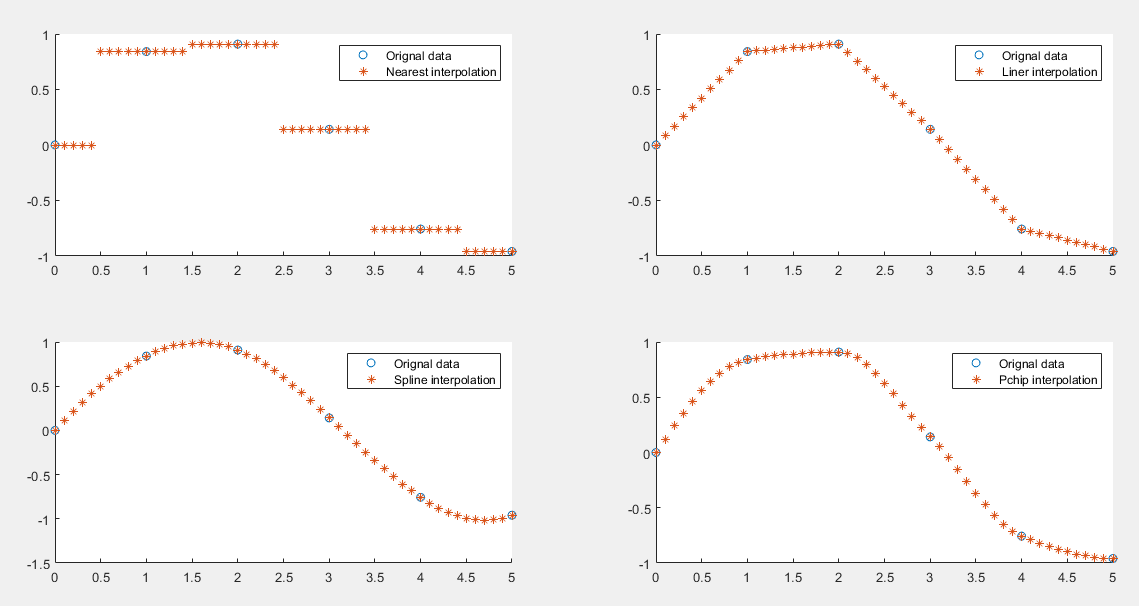• matlab 插值函数
2021-04-23 23:09:42

MATLAB中的插值函数为interp1，其调用格式为：  yi= interp1(x,y,xi,'method')

其中x，y为插值点，yi为在被插值点xi处的插值结果；x,y为向量， 'method'表示采用的插值方法，MATLAB提供的插值方法有几种： 'method'是最邻近插值， 'linear'线性插值； 'spline'三次样条插值； 'cubic'立方插值．缺省时表示线性插值

注意：所有的插值方法都要求x是单调的，并且xi不能够超过x的范围。

例如：在一 天24小时内，从零点开始每间隔2小时测得的环境温度数据分别为

12，9，9，1，0，18 ，24，28，27，25，20，18，15，13，

推测中午12点(即13点)时的温度．

x=0:2:24;

y=[12   9   9   10   18  24   28   27   25   20  18  15  13];

a=13;

y1=interp1(x,y,a,'spline')

结果为：  27.8725

若要得到一天24小时的温度曲线，则：

xi=0:1/3600:24;

yi=interp1(x,y,xi, 'spline');

plot(x,y,'o' ,xi,yi)

更多相关内容
• %interp1对sin函数进行分段线性插值，调用interp1的时候，默认的是分段线性插值 y1=interp1(x,y,xx); figure plot(x,y,'o',xx,y1,'r') title('分段线性插值') %临近插值 y2=interp1(x,y,xx,'nearest'); figure plot...

### 插值

x=0:2*pi;
y=sin(x);
xx=0:0.5:2*pi;

%interp1对sin函数进行分段线性插值，调用interp1的时候，默认的是分段线性插值
y1=interp1(x,y,xx);
figure
plot(x,y,'o',xx,y1,'r')
title('分段线性插值')

%临近插值
y2=interp1(x,y,xx,'nearest');
figure
plot(x,y,'o',xx,y2,'r');
title('临近插值')

%球面线性插值
y3=interp1(x,y,xx,'spline');
figure
plot(x,y,'o',xx,y3,'r')
title('球面插值')

%三次多项式插值法
y4=interp1(x,y,xx,'cubic');
figure
plot(x,y,'o',xx,y4,'r');
title('三次多项式插值')

方法

说明

连续性

注释

'linear'

线性插值。在查询点插入的值基于各维中邻点网格点处数值的线性插值。这是默认插值方法。

C0

• 需要至少 2 个点。

• 比最近邻点插值需要更多内存和计算时间。

'nearest'

最近邻点插值。在查询点插入的值是距样本网格点最近的值。

不连续

• 需要至少 2 个点。

• 最低内存要求

• 最快计算时间

'next'

下一个邻点插值。在查询点插入的值是下一个抽样网格点的值。

不连续

• 需要至少 2 个点。

• 内存要求和计算时间与 'nearest' 相同

'previous'

上一个邻点插值。在查询点插入的值是上一个抽样网格点的值。

不连续

• 需要至少 2 个点。

• 内存要求和计算时间与 'nearest' 相同

'pchip'

保形分段三次插值。在查询点插入的值基于邻点网格点处数值的保形分段三次插值。

C1

• 需要至少 4 个点。

• 比 'linear' 需要更多内存和计算时间

'cubic'

用于 MATLAB® 5 的三次卷积。

C1

• 需要至少 3 个点。

• 点必须均匀间隔

• 对于不规则间隔的数据，此方法会回退到 'spline' 插值

• 内存要求和计算时间与 'pchip' 相似

'v5cubic'

与 'cubic' 相同。

C1

'makima'

修正 Akima 三次 Hermite 插值。在查询点插入的值基于次数最大为 3 的多项式的分段函数。为防过冲，已修正 Akima 公式。

C1

• 需要至少 2 个点。

• 产生的波动比 'spline' 小，但不像 'pchip' 那样急剧变平

• 计算成本高于 'pchip'，但通常低于 'spline'

• 内存要求与 'spline' 类似

'spline'

使用非结终止条件的样条插值。在查询点插入的值基于各维中邻点网格点处数值的三次插值。

C2

• 需要至少 4 个点。

• 比 'pchip' 需要更多内存和计算时间

展开全文• 展开查看详情1.1 第二章 函 数 插 值 — Matlab 插值函数2.2 Matlab 插值函数 Matlab 中的插值函数 interp1 % 分段插值(线性， Hermite ，样条) spline % 三次样条插值 更多插值方法见 Curve Fitting Toolbox csape ...

展开查看详情

1.1 第二章 函 数 插 值 — Matlab 插值函数

2.2 Matlab 插值函数 Matlab 中的插值函数 interp1 % 分段插值(线性， Hermite ，样条) spline % 三次样条插值 更多插值方法见 Curve Fitting Toolbox csape % 可以指定边界条件的三次样条插值 ppval 、 fnval % 计算插值函数在给定点的值

3.3 interp1 yh=interp1(x,y,xh) x 为包含插值节点的 n 维向量 y 为函数在插值节点的值，也是 n 维向量 xh 为需要插值点，可以是一个点，也可以是向量 采用 分段线性插值 方法 一维函数插值

4.4 interp1 可指定插值方法： nearest ， linear ， spline ， pchip 缺省为分段 线性插值，即 linear pchip 为分段三次 Hermite 插值 spline 为样条插值，等价于 spline 一维函数 插值： 指定插值方法 yh=interp1(x,y,xh,method)

5.5 interp1 举例 例 ： 函数 f ( x ) = sin( x ) 在 [0,  ] 上的插值 xi=0:pi/5:pi; % 将插值区间分成若干等距小区间 y i =sin(xi); % 插值节点 处的函数值 x h =0:pi/30:pi; % 需要插值的点 y h = interp1 (xi,yi,xh ); % 根据插值函数求出的近似值 plot(xi,yi,.b , xh,yh,s- ) ; y h = interp1 (xi,yi,xh , nearst ); % 用邻近的值近似 y h = interp1 (xi,yi,xh , pchip ); % 三次 Hermite y h = interp1 (xi,yi,xh , spline ); % 三次样条 ex2f1.m

6.6 spline yh=spline(x,y,xh) 三次样条插值 x 为包含插值节点的 n 维向量 y 为函数在插值节点的值，也是 n 维向量 xh 为需要插值的点，可以是一个点，也可以是向量 采用 三次样条插值 方法

7.7 spline 返回一个结构类型的数据 三次样条插值(返回 插值函数的分段表达式) pp=spline(x,y) pp.breaks 插值节点 pp.coefs 插值分段多项式 系数 pp.pieces 多项式个数 pp.order 分段多项式系数个数，即次数 +1 pp.dim 插值维数 计算插值函数在给定点的值，可以使用 ppval 或 fnval yh=ppval(pp,xh) yh=fnval(pp,xh)

8.8 spline 边界条件 若 x 与 y 的长度相等，则边界条件为 : ( not-a-knot ) 若 y 比 x 多 2 个分量，则采用 第一类边界条件 : 即要求插值函数在第二点和倒数第二个点处三阶连续可导

9.9 spline 举例 例 ： 函数 f ( x ) 定义在 [27.7, 30] 上 ，插值节点及函数值如下 ，求 三次样条 插值 S ( x ) ，边界条件 S ’ (27.7)=3.0, S ’ (30)= - 4.0 x 27.7 28 29 30 f ( x ) 4.1 4.3 4.1 3.0 x i =[ 27.7, 28, 29, 30]; % 插值节点 yi=[ 4.1, 4.3, 4.1, 3.0 ]; % 节点 处的函数值 df0=3.0; dfn =-4.0; % 边界条件 pp= spline (xi,[df0, yi, dfn]); xh=27.7:0.1:30; % 需要插值的点 yh= ppval (pp,xh); % 通过插值求得的近似 值 plot(xh,yh,o-); ex2f2.m

10.10 csape pp = csape(x,y,conds) 边界条件由 conds 给出 ： complete ：第一类边界条件(缺省边界条件) not-a-knot ：非扭结 periodic ：周期(第三类)边界条件 ‘ second ’ ：第二类边界条件 variational ：自然边界条件 可以指定边界条件的三次样条插值 csape 属于 Curve Fitting Toolbox 工具箱 f 0 ’ = y (1), f n ’ = y ( n +2) f 0 ’’ = y (1), f n ’’ = y ( n +2)

11.11 csape 举例 x i =[ 27.7, 28, 29, 30]; % 插值节点 yi=[ 4.1, 4.3, 4.1, 3.0 ]; % 节点 处的函数值 df0=3.0; dfn =-4.0; % 边界条件 pp= csape (xi,[df0, yi, dfn]); xh=27.7:0.1:30; % 需要插值的点 yh= fn val (pp,xh ); % 通过插值求得的近似 值 plot(xh,yh, o- );

展开全文• 主要介绍了matlab中二维插值函数interp2的使用详解，具有很好的参考价值，希望对大家有所帮助。一起跟随小编过来看看吧
• matlab插值函数大全_matlab源码.rar
• 函数格式x1 = interp1(t,x,t1,method); 输入参数： t - 原信号时间轴； x - 原信号幅值； t1 - 插值信号时间轴； method - ‘Nearest’邻近点插值；‘Linear’线性插值；‘Spline’三次样条插值；‘Pchip’立方...

### 函数格式 x1 = interp1(t,x,t1,method);

输入参数：

t - 原信号时间轴；

x - 原信号幅值；

t1 - 插值信号时间轴；

method - ‘Nearest’邻近点插值；‘Linear’线性插值；‘Spline’三次样条插值；‘Pchip’立方插值。默认情况为线性插值！

输出参数：

x1 - t1对应的信号幅值；

### 示例

代码

clc
clear

% 原信号
t=0:1:5;
x=sin(t);

% 插值
t1=0:0.1:5;
x1_n = interp1(t,x,t1,'nearest');
x1_L = interp1(t,x,t1,'linear');
x1_s = interp1(t,x,t1,'spline');
x1_p = interp1(t,x,t1,'pchip');

% 图形
figure
subplot(221)
scatter(t,x)
hold on
plot(t1,x1_n,'*')
legend('Orignal data','Nearest interpolation')
subplot(222)
scatter(t,x)
hold on
plot(t1,x1_L,'*')
legend('Orignal data','Liner interpolation')
subplot(223)
scatter(t,x)
hold on
plot(t1,x1_s,'*')
legend('Orignal data','Spline interpolation')
subplot(224)
scatter(t,x)
hold on
plot(t1,x1_p,'*')
legend('Orignal data','Pchip interpolation')

结果展开全文• MSC Patran中基于Matlab插值函数的多场创建.pdf
• ## MATLAB插值函数interp1

万次阅读 多人点赞 2017-04-15 00:51:02
插值法又称“内插法”，是利用函数f (x)在某区间中已知的若干点的函数值，作出适当的特定函数，在区间的其他点上用这特定函数的值作为函数f (x)的近似值，这种方法称为插值法。如果这特定函数是多项式，就称它为...
• ## MATLAB自带插值函数

千次阅读 2020-04-15 18:25:21
MATLAB中的插值函数为interp1，其调用格式为：yi= interp1(x,y,xi,'method')， 其中x，y为初始插值点，xi为给定的插值点，yi为在被插值点xi处的插值结果。 'method'表示采用的插值方法，MATLAB提供的插值方法有...算法
• Matlab插值函数汇总和使用说明 关于一些基本的源程序
• 然后利用Matlab插值函数将文本中相应场数据转换成为与有限元模型需求一致的场数据；再利用VC++将Matlab求得的场数据按照卡片格式写入bdf文件，以实现对多场的批量创建，通过与MSC Patran创建的场的计算结果进行...
• 当我们遇到高维的数据，二维或者三维的数据时，由于数据的不完整，需要预测一些数值，...本资源实现了matlab环境下的径向基插值函数，并且就算插值函数与真实曲线的各范数误差，误差结果证明了径向基插值函数的优越性。
• MATLAB插值函数2012-08-27 16:11:48|分类： Matlab |举报|字号 订阅matlab中常用的差值函数：interp1 ,interplq ,interpft, spline, intep2, interp3, interpN.2012a中有interp1,interp1q, interpft, spline, pchip,...
• MATLAB 内置函数的区别： 1. 快得多2. 扩展边界值而不是返回 NaN 3. 如果坐标正好在现场，它不会看邻居。 为什么这可能相关的示例： interpolate([blah, blah2], [desiredvalue, NaN], blah) 返回所需值而不是 ...
• matlab开发-拉格朗日插值与导数。此函数执行函数及其导数的拉格朗日插值图像处理与计算机视觉
• ## Matlab中插值函数汇总及使用说明

万次阅读 多人点赞 2018-09-12 19:44:52
MATLAB中的插值函数为interp1，其调用格式为： yi= interp1(x,y,xi,'method')  其中x，y为插值点，yi为在被插值点xi处的插值结果；x,y为向量， 'method'表示采用的插值方法，MATLAB提供的插...
• 分段三次Hermite差值多项式 ...三次样条插值（每个子区间上是三次样条差值多项式） p = spline(x,y,new_x) n维数据插值 p = interpn(x1,x2,…,xn,y,new_x1,new_x2,…,new_xn,method) method是要插值的方法，...
• MATLAB中的插值函数为interp1，其调用格式为： yi= interp1(x,y,xi,'method')其中x，y为插值点，yi为在被插值点xi处的插值结果；x,y为向量， 'method'表示采用的插值方法，MATLAB提供的插值方法有几种： 'method'是...
• 该算法在函数 f 的参数为 x 时确定函数 f，对于 x_L、x_C 和 x_R 的参数，函数的给定值分别为 f_L、f_C 和 f_R。
• matlab三次样条插值函数代码使用基于字典的稀疏表示法对光学相干层析成像中的饱和伪像进行修复 本文介绍的代码： 使用基于字典的稀疏表示法对光学相干断层扫描中的饱和伪像进行修补。 本文介绍了一种基于稀疏表示的...
• ## matlab中的插值函数

千次阅读 2018-12-27 14:10:52
2、interp2(X,Y,Z,Xi,Yi)二维插值（这两种插值函数要求X,Y为单调向量或矩阵） 3、griddata(X,Y,Z,Xi,Yi)二维插值，数据点可以随机选取 4、scatteredinterpolant(X,Y,Z,Xi,Yi)二维插值 其中1和2在大部分书籍和资料...
• matlab三次样条插值函数代码三次样条插值 这是一个函数，用于显示插值的工作方式以及如何将MATLAB inperp2（'spline'）转换为C ++。 关于三次样条的重要说明 当指定样条标记时，MATLAB的interp1假定端点条件不是knot...
• ## MATLAB插值

千次阅读 2021-04-18 04:43:24
在信号处理和图形分析中，插值运算的应用较为广泛，MATLAB提供了多种插值函数，可以满足不同的需求。4.5.1 一维数据插值一维数据插值常使用函数interp1，其一般的语法格式为：yi=interp1(x,y,xi,method)。其中y为函...
• 方法一：Lagrange插值 function [y]=lagrange(x0,y0,x) n=length(x0);y=zeros(1,length(x)); for k=1:n t=ones(1,length(x)); for i=1:n if i~=k t=t.*(x-x0(i))/(x0(k)-x0(i)); end end y=y+t*y...
• 基于matlab的分段线性插值实现、分段三次样条插值、分段四次样条插值(不使用matlab自带函数)
• ## Matlab插值

千次阅读 2020-07-07 14:57:08...

# matlab插值函数matlab 订阅